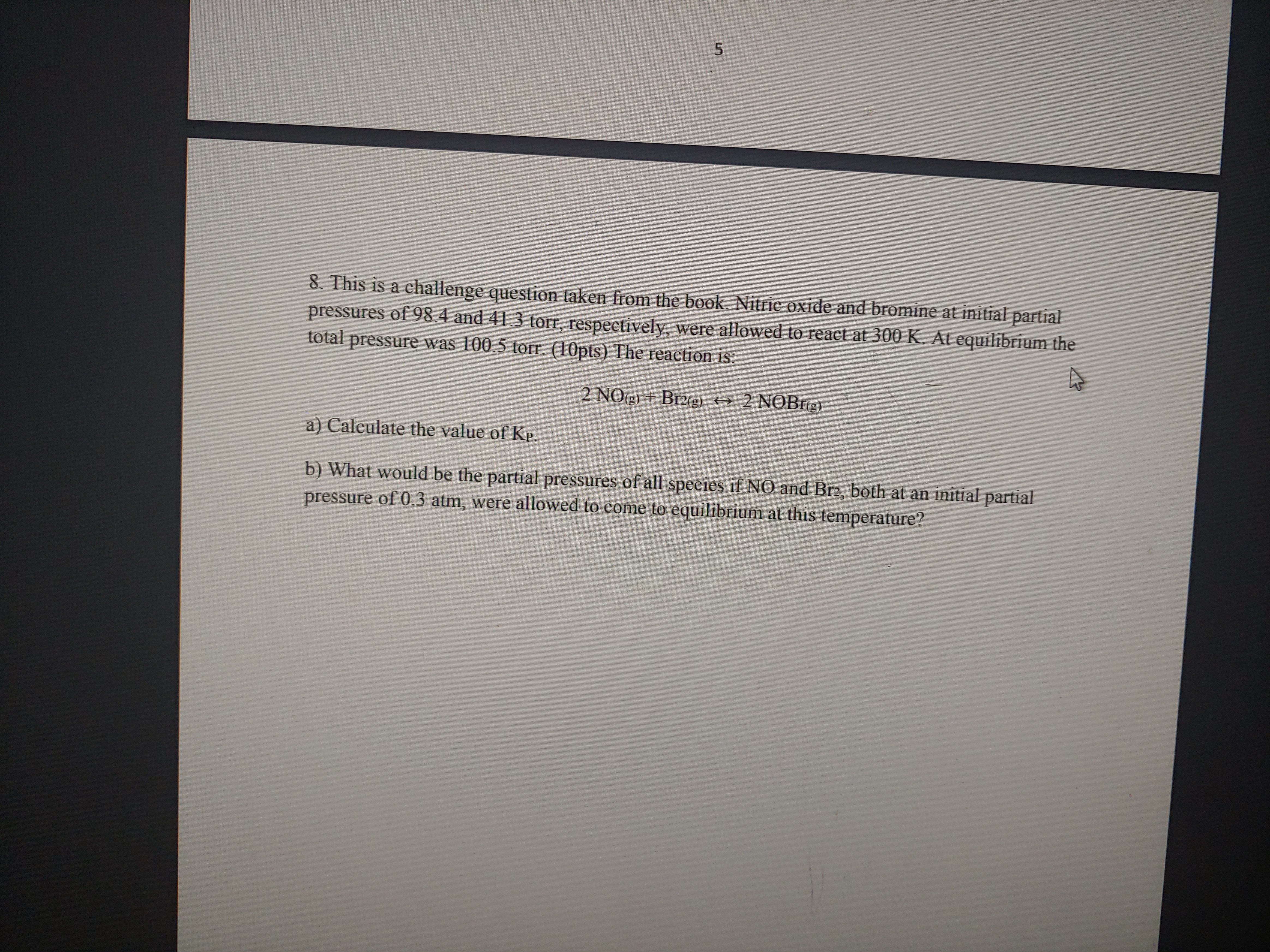# Nitric oxide and bromine at initial partial pressures of 98.4 and 41.3 torr, respectively, were allowed to react at 300 K. At equilibrium, the total pressure was 100.5 torr. The reaction is: 2 NO (g) + Br2 (g) <= > 2NOBr (g) a) Calculate the value of Kp